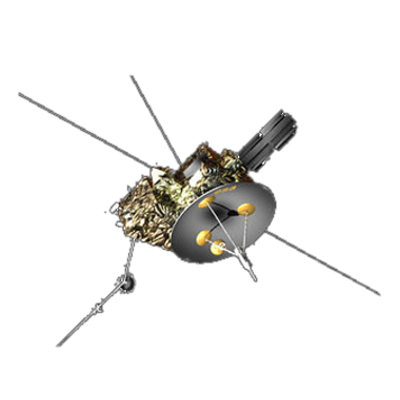ULYSSES

Ulysses HISCALE Data Analysis Handbook

### 4.11 Calculated Responses of HISCALE Sensors to Ulysses RTG Radiation

Document source: Juan Gomez, University of Kansas
Date: 4/19/96

4.11.1 Introduction

We are interested in the g-ray response of silicon detectors on Ulysses. Specifically, we want to produce a reasonable energy loss spectrum from RTG.

We compare the simulated spectra with the measured spectra from some selected "quiet" times, and determine if the background is a result of RTG.

Although there are several methods used to simulate detector performance , we will use a Monte Carlo type algorithm, which is well suited to simulate the natural stochastic processes in gamma ray interactions .

4.11.2 Method

When penetrating a silicon detector, g-rays can interact with the atoms in various ways. The two processes we must consider are:

1.) Compton scattering
2.) pair production

Using the interaction cross sections, sc and sp-p, one can obtain the probability that each interaction will occur . The type of interaction a photon undergoes is then randomly determined, consistent with the relative probabilities of the interactions at the specified energy.

Once the type of interaction is determined, we must then generate a random interaction path length, based on the mean free path of the photon for the interaction and the interaction cross section. We then check the position of the photon to see if the interaction length will take it out of the detector. This process is then repeated until the photon leaves the detector, or until pair production has occurred. For the dimensions of the silicon detectors used in this study, a photon will undergo at most 2-3 interactions before leaving the detector. The method for tracking the photon inside the detector will be explained in more detail in the next section.

For each photon that interacts with the detector, we add up the total energy deposited inside the detector by the secondary electrons . This quantity is then "binned" in order to simulate the detector response.

4.11.3 The Simulation

We run the simulation for two cases:

1. incidence direction of RTG photons along the axis of the detector
2. incidence direction of RTG photons at 90° to the axis

We use the following RTG spectrum :

 # of Photons Energy (MeV) 3600 2.45 220 1.87 390 1.62 320 1.37 460 1.12 2900 0.87 4000 0.61 950 0.45 460 0.37 520 0.32 270 0.28 1300 0.23 300 0.18 700 0.16 370 0.14 255 0.11 330 0.07

Next, we determine how the photon behaves when it reaches the detector. This involves a multi-step process:

1. Determine a unit vector corresponding to the direction of approach of the incoming photon .
2. Using sc and sp-p, determine the interaction process.
3. Using s and lmfp, determine x, the distance the photon travels before interacting.
4. Advance the photon by x inside the detector and check to see if it leaves the detector. If it leaves the detector then go to step 1.
5. If the photon remains inside the detector then:
1. if the process is Compton:

(i) calculate the Compton angle qc via the Klein-Nishina formula, and calculate the secondary electron energy .
(ii) update the unit vector of the secondary photon (it now becomes the new "incident" photon) and go to step 3.

2. if the process is pair production:

(i.) calculate the energy of the secondary electron .
(ii.) assume that no reactions will occur after pair production, and go to step 1.

6. After each photon has completed the simulation, bin the total energy of the secondary electrons to obtain the energy spectra.

The results for the LEMS and LEFS detectors are shown in Figures 4.79 through 4.82.

Figures:

• Figure 4.79 Comparison of simulation results to S/C data - LEMS 120
• Figure 4.80 Comparison of simulation results to S/C data - LEMS 30
• Figure 4.81 Comparison of simulation results to S/C data - LEFS 60
• Figure 4.82 Comparison of simulation results to S/C data - LEFS 150
• Figure 4.83 Flow chart for RTG simulation

4.11.4 Conclusions

1.) Normalization has not been done.

2.) Shape from RTG axial incidence g's is similar to shape observed in quiet times.

3.) RTG radiation could account for observations if the source intensity is adjusted.

A single adjustment could be made for each telescope for distance and another for orientation.

References:

 R. Bell, Nucl. Inst. and Meth., 93, p. A-2 (1971)
 M.Kalos and P. Whitlock, Monte Carlo Methods, pp 129-144, Wiley (1986)
 A. Beiser, Concepts of Modern Physics, Ch.12, McGraw-Hill (1981)
 K. Krane, Introductory Nuclear Physics, Ch. 7, Wiley (1988)
 R.W. Campbell, ISPM RTG Simulation (1980)